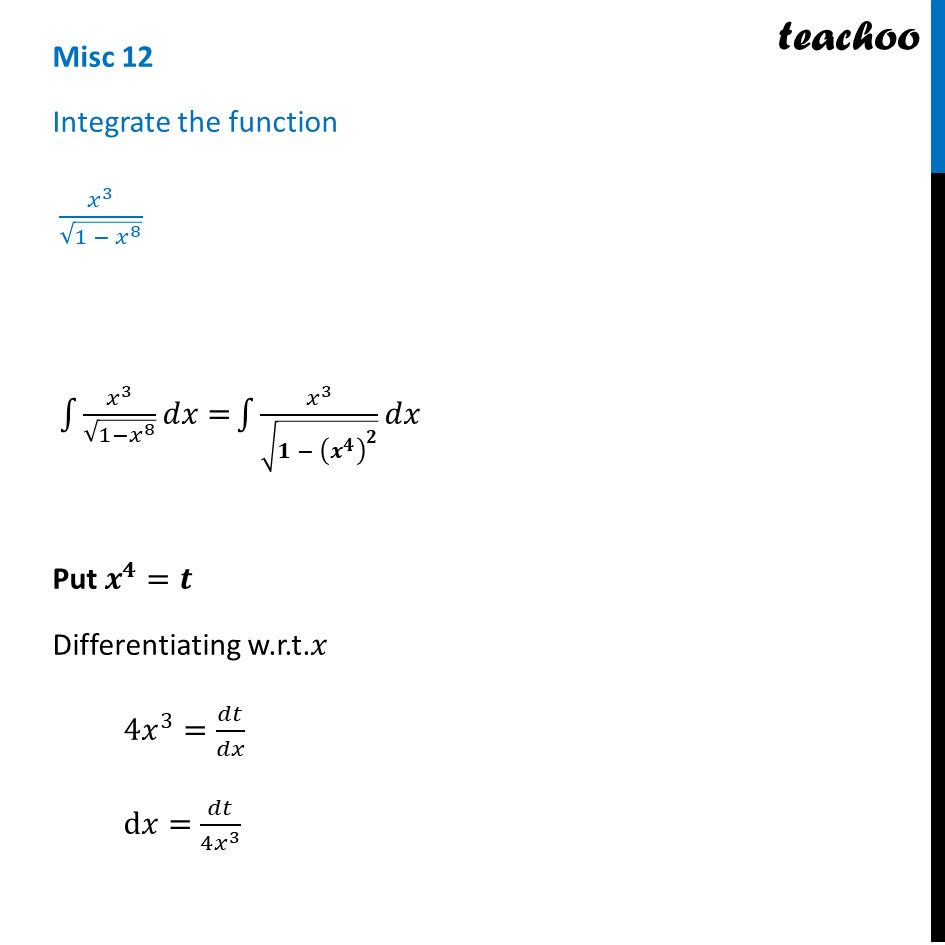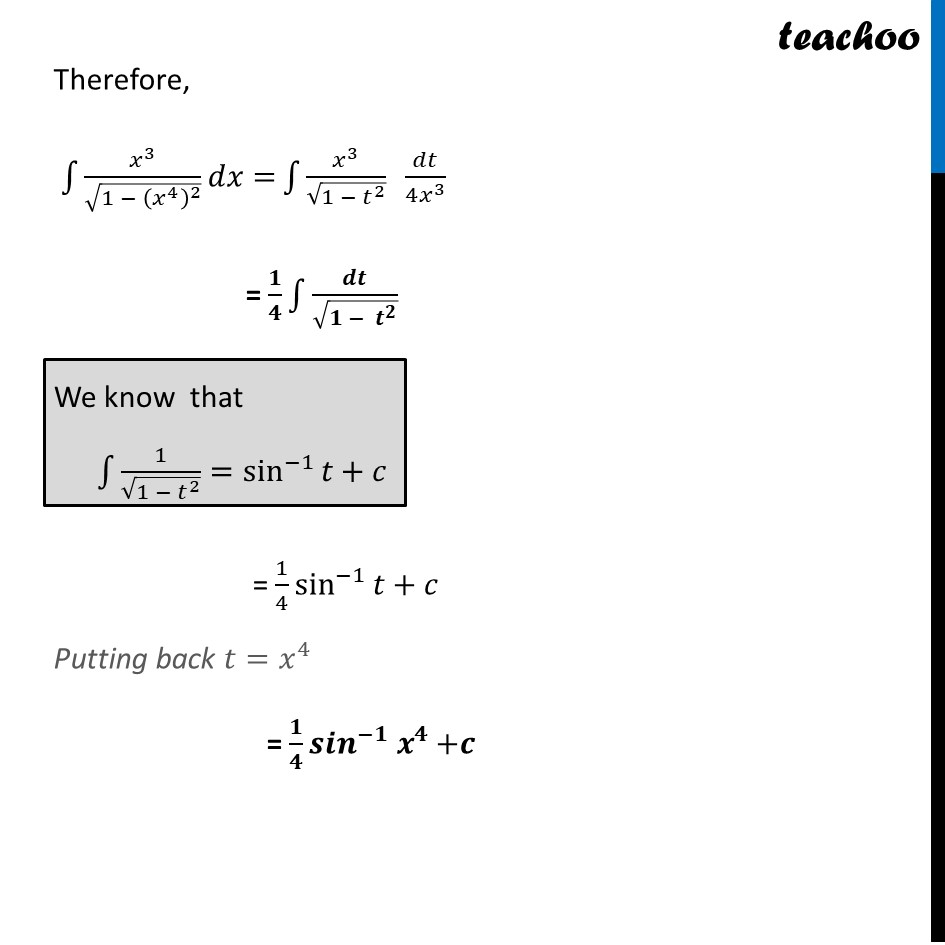Integration by specific formulaes - Formula 5

Chapter 7 Class 12 Integrals
Concept wiseLearn in your speed, with individual attention - Teachoo Maths 1-on-1 Class

### Transcript

Misc 12 Integrate the function 3 1 8 3 1 8 = 3 1 4 2 Put 4 = Differentiating w.r.t. 4 3 = d = 4 3 Therefore, 3 1 4 2 = 3 1 2 4 3 = 1 4 1 2 = 1 4 sin 1 + Putting = 4 = +